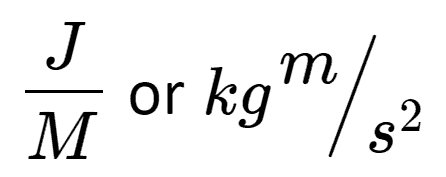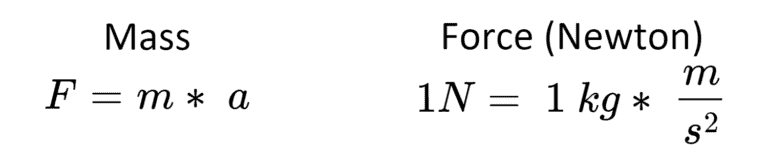# Force Converter

Kilonewtons:
kN
Newtons:
N
Kilogram-Force:
kgf
Gram-Force:
gf
Kilogram Meters/Second2:
kgm/s2
Metric Ton-Force:
tnf
Short Ton-Force (US):
tnf
Long Ton-Force (UK):
tnf
Kilopound-Force:
kipf
Pound-Force:
lbf
Ounce-Force:
ozf
Joule/Meter:
J/m
Joule/Centimeter:
J/cm
Dyne:
dyn
Poundal:
pdl
Sthene:
sn

## dBm to Watts Converter

Kilonewtons:
kN
Newtons:
N
Kilogram-Force:
kgf
Gram-Force:
gf
Kilogram Meters/Second2:
kgm/s2
Metric Ton-Force:
tnf
Short Ton-Force (US):
tnf
Long Ton-Force (UK):
tnf
Kilopound-Force:
kipf
Pound-Force:
lbf
Ounce-Force:
ozf
Joule/Meter:
J/m
Joule/Centimeter:
J/cm
Dyne:
dyn
Poundal:
pdl
Sthene:
sn

Suntsu’s force converter will help you convert quickly between different units of force. Force can best be defined as the interaction that will change the motion of an object. In other words, Force is a push, pull, or dragging on an object that changes its velocity or direction. The unique quality of force is that it is an arbitrary measurement, meaning “1 unit” is set by the person assigning it and all other measures follow suit. Suntsu’s converter has the following units:

Newton (N) orGram-Force (gf)

Metric Ton-Force

Short Ton-Force (US)

Long Ton-Force (UK)

Sthène (sn)

Pound-Force (lbf)

Ounce-Force (ozf)

Dyne (dyn)

Poundal (pdl)

The universal measure of force is Newtons. Designated that name after Isaac Newton, Newtons originate from his second law of motion. The law is as follows; the force applied (F) is equal to the mass (m) of the object receiving the force multiplied by the acceleration (a) of the object. A newton is equal to the force needed to accelerate one kilogram of mass at the rate of one meter per second squared in the direction that force is applied.Although Newtons are the SI unit of force there are others to consider. Dynes used to be the universal SI unit before Newtons came along. A Dyne is defined as the force needed to accelerate a mass of 1 gram at a rate of one centimeter per second squared. One Dyne is equal to 0.00001 Newton. Although this unit is hardly used today, it works well with small objects.

A gram-force, another unit of measurement of force, is equal to a mass of one gram multiplied by the standard acceleration due to gravity. One newton is equal to 101.97 gram-force. Additionally, there is metric ton-force which is one of many units of force defined as the weight of one ton due to gravity. A metric ton is 1000kg, resulting in one metric ton-force equaling to 9806.65 newtons. In Suntsu’s converter, we also include short ton-force and long ton-force. A short ton is a US ton that is measured at 2000 pounds, as a result, short ton-force if the force of Earth’s gravity acting on a short ton. One short ton-force is equal is 8896.4432 newtons. Conversely, a British ton is the long ton measured at 2240 pounds, as a result, long ton-force is the force of gravity acting on a long ton. One long ton-force is equal to 9964.0164 newtons. Sthène, an obsolete unit of force in the meter-ton-second system, is the same as one kilonewton or 0.001 newtons.

A poundal, as part of the foot-pound-second system, is defined as the force necessary to accelerate 1 pound-mass at 1 foot per second. One poundal approximately equal to 0.138255 newton. An additional unit of the foot-pound-second system is the pound-force. Not to be confused with a foot-pound which is a unit of energy, a pound-foot is equal to the gravitational force exerted on a mass of one avoirdupois pound (based on 16 ounces) on the surface of the Earth. One newton is approximately equal to 0.22581 pound-force.

Newton (N) orGram-Force (gf)

Metric Ton-Force

Short Ton-Force (US)

Long Ton-Force (UK)

Sthène (sn)

Pound-Force (lbf)

Ounce-Force (ozf)

Dyne (dyn)

Poundal (pdl)

The universal measure of force is Newtons. Designated that name after Isaac Newton, Newtons originate from his second law of motion. The law is as follows; the force applied (F) is equal to the mass (m) of the object receiving the force multiplied by the acceleration (a) of the object. A newton is equal to the force needed to accelerate one kilogram of mass at the rate of one meter per second squared in the direction that force is applied.Although Newtons are the SI unit of force there are others to consider. Dynes used to be the universal SI unit before Newtons came along. A Dyne is defined as the force needed to accelerate a mass of 1 gram at a rate of one centimeter per second squared. One Dyne is equal to 0.00001 Newton. Although this unit is hardly used today, it works well with small objects.

A gram-force, another unit of measurement of force, is equal to a mass of one gram multiplied by the standard acceleration due to gravity. One newton is equal to 101.97 gram-force. Additionally, there is metric ton-force which is one of many units of force defined as the weight of one ton due to gravity. A metric ton is 1000kg, resulting in one metric ton-force equaling to 9806.65 newtons. In Suntsu’s converter, we also include short ton-force and long ton-force. A short ton is a US ton that is measured at 2000 pounds, as a result, short ton-force if the force of Earth’s gravity acting on a short ton. One short ton-force is equal is 8896.4432 newtons. Conversely, a British ton is the long ton measured at 2240 pounds, as a result, long ton-force is the force of gravity acting on a long ton. One long ton-force is equal to 9964.0164 newtons. Sthène, an obsolete unit of force in the meter-ton-second system, is the same as one kilonewton or 0.001 newtons.

A poundal, as part of the foot-pound-second system, is defined as the force necessary to accelerate 1 pound-mass at 1 foot per second. One poundal approximately equal to 0.138255 newton. An additional unit of the foot-pound-second system is the pound-force. Not to be confused with a foot-pound which is a unit of energy, a pound-foot is equal to the gravitational force exerted on a mass of one avoirdupois pound (based on 16 ounces) on the surface of the Earth. One newton is approximately equal to 0.22581 pound-force.